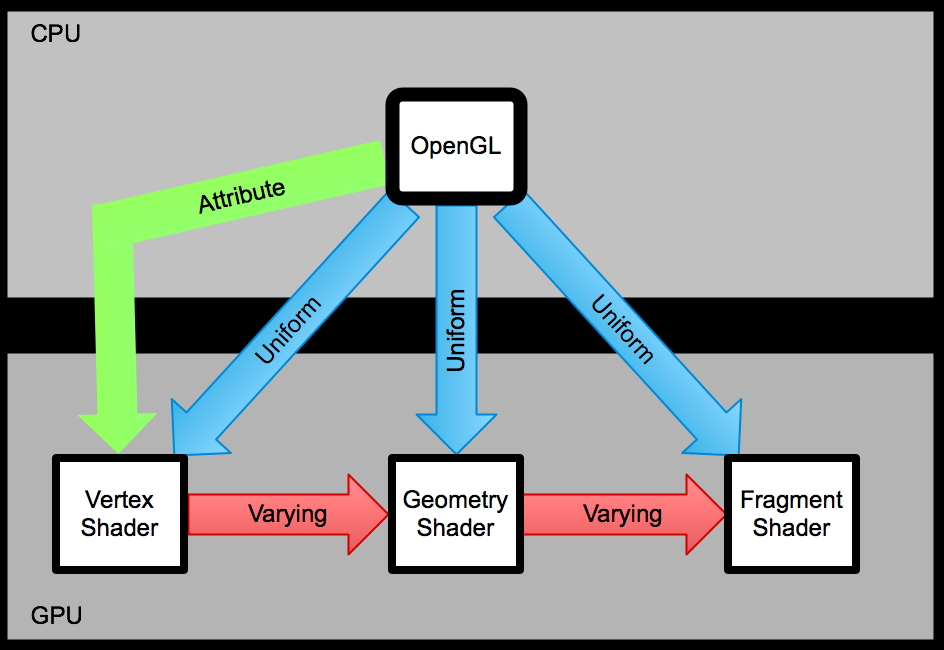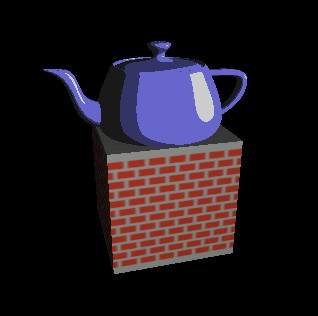# Week 2

## GLSL langauge study

(notes from the lighthouse3d tutorial and the Orange book)

Data Types

int
float
bool

vec{2,3,4} a vector of 2, 3,or 4 floats
bvec{2,3,4} bool vector
ivec{2,3,4} vector of integers

mat2 - 2x2 matrix
mat3 - 3x3 matrix
mat4 - 4x4 matrix

sampler1D - abstraction for looking up values in 1D textures
sampler2D - ditto for 2D textures
sampler3D - ditto for 3D textures
and a few more

arrays - similar to C

structures - similar to C

no pointers
no casting, but you have int() and float() functions

Variables:

can do initialization when declaring a variable as long as the type is correct:
int a = 2;
float b = 2.0;
bool c = true;
vec4 d = vec4(1.0, 2.0, 3.0, 4.0);

can access vector components more easily: xyzw and rgba, and stpq
float xPos = d.x
vec3 e = d.rgb
vec2 p = d.xy

d == d.y == d.g == d.t

• const
• compile time constant
• attribute
• global variable (at the top level of the shader code)
• can change per vertex (within a glBegin/glEnd)
• passed in from OpenGL program
• only used in vertex shaders
• uniform
• global variable (at the top level of the shader code)
• can change per primitive (not within a glBegin/glEnd)
• passed in from OpenGL program
• used in vertex, geometry, and fragment shaders
• varying
• used to pass data from a vertex shader to a fragment shader (or vertex to geometry, geometry to fragment)
• describe attributes that vary across a primitive
• declarations must match in the two connected shaders (vert and geom, vert and frag, geom and frag)
• gets per-vertex values in the vertex shader which are then automatically interpolated into a value for the current fragment in the fragment shaderFor a single primitive
• vertex shader runs once per vertex
• fragment shader runs once per fragment
• geometry shader runs once per primitive
• multiple instances of the same shader may run in parallel - order is unknown
There is no communication from vertex to vertex or fragment to fragment or geometry to geometry

Statements and Functions

if (boolean expression)
{}
else
{}

and possibly:

for (init; boolean expression; loop expression)
{}

while (boolean expression)
{}
do

do
{}
while (boolean expression)

The vertex shader, geometry shader, and the fragment shader must have a single main function - typically void main()

you can also write your own functions with return values to help main do its job

Communication between OpenGL and GLSL:

How do you communicate with a shader:
• Read only user defined variables
• Uniform Variables
• Attribute Varriables
• Textures

Built-in Uniform Variables

OpenGL state - light properties

struct gl_LightSourceParameters {
vec4 ambient;
vec4 diffuse;
vec4 specular;
vec4 position;
vec4 halfVector;
vec3 spotDirection;
float spotExponent;
float spotCutoff; // (range: [0.0,90.0], 180.0)
float spotCosCutoff; // (range: [1.0,0.0],-1.0)
float constantAttenuation;
float linearAttenuation;
};

uniform gl_LightSourceParameters gl_LightSource[gl_MaxLights];

struct gl_LightModelParameters {
vec4 ambient;
};

uniform gl_LightModelParameters gl_LightModel;

which allows us to access things like gl_LightSource.position

OpenGL state - material properties

struct gl_MaterialParameters {
vec4  emission;
vec4  ambient;
vec4  diffuse;
vec4  specular;
float shininess;
};

uniform gl_MaterialParameters gl_FrontMaterial;
uniform gl_MaterialParameters gl_BackMaterial;

OpenGL state - and a few more

uniform   mat4  gl_ModelViewMatrix;
uniform   mat4  gl_ProjectionMatrix;
uniform   mat4  gl_ModelViewProjectionMatrix;
uniform   mat3  gl_NormalMatrix;
uniform   mat4  gl_TextureMatrix[n];

(there is a full list on pages 105-107 of the Orange Book V2) and more info on this in Chapter 4 in general

Uniform Variables
- value can be changed, but not within a glBegin glEnd pair
- can be read but not written within vertex, geometry, and fragment shaders
- program must be linked before trying to get at the memory location

- within the application you need to get the memory location of the variable
GLint glGetUniformLocation(GLuint program, const char *name);

uniform vec3 LightPos;

in the application:
GLint lightLoc;
lightLoc = glGetUniformLocation(p, "LightPos"); // assuming program p

- then you can assign values to it:
GLint glUniform{1|2|3|4}{if}(GLint location, TYPE value);
GLint glUniform{1|2|3|4}{if}v(GLint location, GLsizei count, const TYPE *values);

e.g. glUniform3f(lightLoc, 1.0, 1.0, 4.0);

for booleans you can use either int or float version (0=false, anything else=true)

GLint glUniformMatrix{2|3|4}fv(GLint location, GLsizei count,
GLboolean transpose, const GLfloat *values);

- values are set until program is linked again

Vertex Shader: (so you know where normal and lightDir are coming from)

varying vec3 normal, lightDir;

void main()
{
vec4 p;

// the light position is already stored in eye space coordinates
lightDir = normalize(vec3(gl_LightSource.position));

// convert normal to eye space coordinates using gl_NormalMatrix
// gl_NormalMatrix is built in uniform matrix provided by GLSL
// representing the inverse transpose model-view matrix
normal = normalize(gl_NormalMatrix * gl_Normal);

gl_Position = ftransform();
}

uniform float specIntensity;
uniform vec4  specColor;
uniform float t;
uniform vec4  colors;

// all of the uniform variables could have been defined within the shader
// but this way we get to pass those values in from the OpenGL program

varying vec3  normal, lightDir; // must match those in the vertex shader

void main()
{
float intensity;
vec3 n;
vec4 color;

n = normalize(normal);
intensity = max(dot(lightDir, n),0.0);

// intensity tells me how perpendicular the light is at this point

if (intensity > specIntensity)
color = specColor;
else if (intensity > t)
color = colors;
else if (intensity > t)
color = colors;
else
color = colors;

gl_FragColor = color;
}

OpenGL application

(in void setShaders() in the typical examples after reading, compiling, attaching, linking, and using the shaders ... just before the glut main loop gets called):

GLint loc1,loc2,loc3,loc4;
float specIntensity = 0.98;
float sc = {0.8, 0.8, 0.8, 1.0};
float threshold = {0.5, 0.25};
float colors = {0.4, 0.4, 0.8, 1.0,
0.2, 0.2, 0.4, 1.0,
0.1, 0.1, 0.1, 1.0};

loc1 = glGetUniformLocation(p, "specIntensity");
glUniform1f(loc1, specIntensity);

loc2 = glGetUniformLocation(p, "specColor");
glUniform4fv(loc2,1,sc);
//or glUniform4f(loc2,sc,sc,sc,sc);

loc3 = glGetUniformLocation(p, "t");
glUniform1fv(loc3,2,threshold);

loc4 = glGetUniformLocation(p, "colors");
glUniform4fv(loc4,3,colors);

void renderScene(void) {
glClear(GL_COLOR_BUFFER_BIT | GL_DEPTH_BUFFER_BIT);

gluLookAt(0.0,0.0,5.0,
0.0,0.0,-1.0,
0.0f,1.0f,0.0f);

glLightfv(GL_LIGHT0, GL_POSITION, lpos);
glRotatef(a,0,1,0);
glutSolidTeapot(1);
a++;

glutSwapBuffers();
}

Which gives us a toon shaded teapot rotating horizontally like this:

The main code for this is here in ogl2.cpp

There are some more tutorials with the teapot at lighthouse3d that may be worth looking into:
http://www.lighthouse3d.com/opengl/glsl/index.php?toon

Attribute Variables

- value can be changed within a glBegin glEnd pair
- not useful in a fragment shader
- within the application  you need to get the memory location of the variable
GLint glGetAttribLocation(GLuint program, const char *name);

- then you can assign values to it:
GLint glVertexAttrib{1|2|3|4}{s|f|d}ARB(GLint index, TYPE v);
GLint glVertexAttrib{1|2|3|4}{s|f|d}vARB(GLint index, const TYPE *v);

e.g. glVertexAttrib1f(loc,2.0);

built-in ones:
attribute vec4  gl_Color;
attribute vec4  gl_SecondaryColor;
attribute vec4  gl_Normal;
attribute vec4  gl_Vertex;
attribute vec4  gl_MultiTexCoord0;
attribute float gl_FogCoord;

example:

GLint loc;

(in void setShaders() in the typical examples after reading, compiling, attaching, linking, and using the shaders ... just before the glut main loop gets called):

loc = glGetAttribLocation(p,"height");

float a=0;

void renderScene(void)
{
glClear(GL_COLOR_BUFFER_BIT | GL_DEPTH_BUFFER_BIT);

gluLookAt(0.0,0.0,10.0,
0.0,0.0,-1.0,
0.0f,1.0f,0.0f);

glLightfv(GL_LIGHT0, GL_POSITION, lpos);
glRotatef(a,0,1,0);

glBegin(GL_TRIANGLE_STRIP);
glVertexAttrib1f(loc, 2.0);
glVertex2f(-1,1);
glVertexAttrib1f(loc, 2.0);
glVertex2f(1,1);
glVertexAttrib1f(loc,-2.0);
glVertex2f(-1,-1);
glVertexAttrib1f(loc,-2.0);
glVertex2f(1,-1);
glEnd();

a+=0.1;

glutSwapBuffers();
}

attribute float height;

void main()
{
vec4 p;

// gl_Vertex is an attribute variable provided by GLSL
p.xz = gl_Vertex.xy;
p.y  = height;
p.w  = 1.0;

// gl_ModelViewProjectionMatrix is built in uniform matrix provided by GLSL
// we're not just passing through the vertex information untouched this time
gl_Position = gl_ModelViewProjectionMatrix * p;
}

void main()
{
// make everything greenish
gl_FragColor = vec4(0.4,0.8,0.4,1.0);

}

Without the vertex shader we would have a square (-1 to 1 in two dimensions) spinning around the vertical axis through its center as shown in the movie below on the left. The shader takes the 2D vertex given and converts it to a 3D vertex (x->x, y->z, height becomes y)
which gives us a green rectangle rotating horizontally as shown in the movie below on the right.

The main code for this is here in ogl3.cpp

There is a related flatten shader tutorial at lighthouse3d that may be worth looking into:
void main(void)
{
vec4 v = vec4(gl_Vertex);
v.z = 0.0;

gl_Position = gl_ModelViewProjectionMatrix * v;
}

Built-in Varying Variables

varying vec4 gl_FrontColor;
varying vec4 gl_BackColor;
varying vec4 gl_FrontSecondaryColor;
varying vec4 gl_BackSecondaryColor;
varying vec4 gl_TexCoord[gl_MaxTextureCoords];
varying vec4 gl_FogFragCoord;

varying vec4 gl_Color;
varying vec4 gl_SecondaryColor;

Built-in Functions:

Trig Functions

• degrees
• sin
• cos
• tan
• asin
• acos
• atan

Exponential Functions:
• pow
• exp2
• log2
• sqrt
• inversesqrt

Common Functions:

• abs
• sign
• floor
• ceil
• fract
• mod
• min
• max
• clamp
• mix (x, y, a) x*(1.0 - a) + y*a
• step (edge, x) x <= edge ? 0.0 : 1.0
• smoothstep (edge0, edge1, x) we will look at this next time

Geometric Functions:
• length
• distance
• dot
• cross
• normalize
• ftransform
• faceforward
• reflect
• refract

Matrix Functions:
• matrixcompmult

Vector Relational Functions:
• lessThan
• lessThanEqual
• greaterThan
• greaterThanEqual
• equal
• notEqual
• any
• all
• not
Texture Access Functions:

Fragment Processing Functions:

Noise Functions:

One important thing to note is that it can be tricky to debug shaders. You can't just add a printf() and see what's happening. What you can do is check the info log using functions like this from www.lighthouse3d.com:

{
GLint infoLogLength = 0;
GLsizei charsWritten  = 0;
GLchar *infoLog;

if (infoLogLength > 0)
{
infoLog = (char *) malloc(infoLogLength);
printf("%s\n",infoLog);
free(infoLog);
}
}

void printProgramLog(GLuint obj)
{
GLint infoLogLength = 0;
GLsizei charsWritten  = 0;
GLchar *infoLog;

glGetProgramiv(obj, GL_INFO_LOG_LENGTH, &infoLogLength);

if (infoLogLength > 0)
{
infoLog = (char *) malloc(infoLogLength);
glGetProgramInfoLog(obj, infoLogLength, &charsWritten, infoLog);
printf("%s\n",infoLog);
free(infoLog);
}
}

and then you can call these functions like this:

p = glCreateProgram();

printProgramLog(p);

which will give errors like this if you mistype something within the shader code:
ERROR: 0:25: '/' : syntax error syntax error
ERROR: Parser found no code to compile in source strings.

ERROR: One or more attached shaders not successfully compiled

Thursday:

Go through the brick shader tutorial that we briefly showed in Lecture 1, which is Chapter 6 of the Orange Book

It is also available on-line here:

http://www.awprofessional.com/articles/article.asp?p=171029&seqNum=1